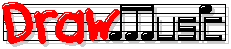Pop Music Theory

Lessons

Detailed Contents

26: Diatonic Function Analysis

Get Future Lessons

Detailed Contents

# Lesson 26: Diatonic Function Analysis

This lesson teaches how to understand the diatonic chords in "real" songs.

Before taking this lesson, you should know: the diatonic chord functions (Lesson 25: Subdominant & Dominant).

To learn to use the diatonic chord functions (tonic, subdominant, and dominant), you should study them in real songs. This is called diatonic function analysis.

Let's do an example. Here's a short chord progression in the key of C major (it's actually a simplified phrase from Bob Dylan's Like A Rolling Stone):

 C Dm Em F G C

First, add Roman numeral symbols (from Lesson 23: Using Diatonics):

 I IIm IIIm IV V I C Dm Em F G C

Next, we'll add diatonic function symbols. We'll use these symbols:

 T = Tonic (T) = "substitute" Tonic SD = Subdominant D = Dominant

Here's the example with the diatonic functions added:

 Functions: T SD (T) SD D T Roman symbols: I IIm IIIm IV V I Actual chords: C Dm Em F G C

## Tension Levels

The diatonic functions have different tension levels, which means how at rest, or not at rest, that function feels. Here are the tension levels, from "highest tension" to lowest:

 D (Dominant): Highest tension; "really wants to" return to Tonic. SD (Subdominant): Not at rest. Often used to move away from Tonic; often moves to Dominant. (T) (substitute Tonic): Feels "semi" at rest. T (Tonic): Feels at rest.

The way a chord progression moves among the tension levels creates that progression's tension story. Here's our example progression (C → Dm → Em → F → G → C) again, displayed as a tension-level graph so you can see the tension story's shape:

 C Dm Em F G C D V SD IIm IV (T) IIIm T I I

Following the Roman symbols above from left to right, we can see that this example's basic tension story is:
1. Starts at rest, on I
2. Climbs (with a bit of wandering) to high tension (the V)
3. Drops back to rest (the last I)

This particular story/shape is very, very common, but there are lots of variations and alternatives. To develop a feel for the effects of different tension stories, you should include diatonic function analysis when you're analyzing songs (see Lesson 2: Practicing Songwriting). Make tension-level graphs for lots of different songs, make up tension stories from the graphs, and decide for yourself what you think the musical effects of different tension stories are.

Next: